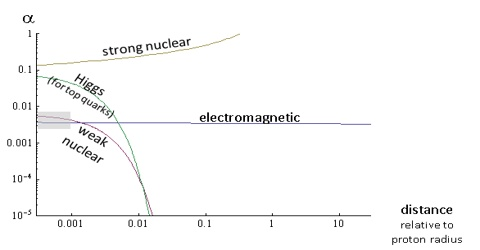# Comparison of Intensities of the Fundamental Force

Comparison of intensities of the fundamental force

Comparison of relative strength of the four fundamental forces shows that strongest force is the strong nuclear force and the weakest one is the gravitational force. There are four fundamental forces: strong, electromagnetic, weak, and gravitational, although the electromagnetic and weak forces can be united into a single so-called electroweak force. In addition, a number of experiments have been performed that hint at the existence of a fifth fundamental force, although none of these has ever been confirmed.

Both strong and weak nuclear forces are short range forces. These forces are not active outside the nucleus. On the other hand range of gravitational and electro-magnetic force is almost infinity.

In order to have an idea about the strength of the four fundamental forces, if we take the value of strong nuclear force as 1 then relative strengths of the weak nuclear force, electromagnetic force and gravitational force are 10-12, 10-2 and 10-39 respectively.

Scientists have been trying for many years to establish relation between the four fundamental forces. Prof. Abdus Salam, Wienberg and Glashow— these three scientists, after many years of research, have established a relation between weak nuclear force and electro-magnetic force which is known as Salam-Wienberg’s Theory.